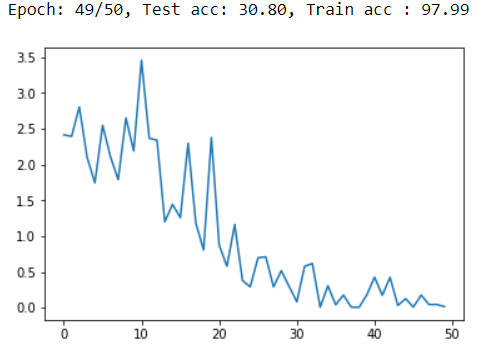I have trained a model using resnet18 from scratch. I save a model with a minimum loss to a .pth file. The training accuracy came around 90 % and testing accuracy around 15%.
On loading the file and calling evaluation(testloader, resent) or evaluation(trainloader, resnet) the percentage keeps varying each time I load the model example on calling evaluation(trainloader, resnet) i would get values like 98% 97% etc. So why does this happen?

Are you calling `model.eval()` on the model before running the evaluation?
This would make sure to use the running stats in batchnorm layers (and not update them anymore) and disable dropout layers.

no i wasnt calling model.eval()

Also have this doubt
Hi
I’m currently working on a classification model whose input are x-ray images, i need to classify it into the respective disease categories. I have trained resnet 18 (not pretrained )on a data-set of 100 images per class.

I have 1493 images out of which 1045 for training and 448 images for testing (70:30 split).

On running for 50 epochs the training accuracy goes to 97.99 percent, but the testing accuracy isn’t good at all it is just about 30 %

this is plotting loss per epochThe testing accuracy is low since there arent sufficient images?

I have grey scale images and i have down-scaled them to 224 x 224 so image shape is [1,224,224].
original image size 1024x1024

I have modified the first conv2d layer to accept grey scale images and last layer of resnet to output the required number of classes.

The general workflow sounds reasonable.
However, I doubt you’ll be able to train a model from scratch with just 1000 images.
You could try to use a pretrained model (even though the pretraining used data from another domain) and compare the results.

Oh okay will try that

Also while plotting confusion matrix there’ s a problem i have only 1493 images in total and 1045 go for training and 448 for testing…but the confusion matrix rows sum up to 5100…what can be the error.

#preds = torch.tensor([])
confusion_matrix = torch.zeros(num_classes, num_classes)
model added as argument - 1 change from lenet
total, correct = 0,0
inputs, labels, imgindex = data
inputs, labels, imgindex = inputs.to(device), labels.to(device), imgindex
# inputs are put in the gpu
outputs = model(inputs)
# from net to model - 2 change from lenet
_, pred = torch.max(outputs.data,1)
for t, p in zip(labels.view(-1), pred.view(-1)):
confusion_matrix[t.long(), p.long()] +=1
total += labels.size(0)
correct +=(pred==labels).sum().item()
return 100 * correct/total

this is the evaluation function

Also what should be the weight and SD for grey scale images for using pretrained resnet 18?

Not sure, what’s causing the higher number of samples in your confusion matrix, but the code seems to be fine generally:

``````m = torch.zeros(10, 10)

pred = torch.randint(0, 10, (100,))
target = torch.randint(0, 10, (100,))

for t, p in zip(target.view(-1), pred.view(-1)):
m[t.long(), p.long()] += 1

print(m.sum())
> tensor(100.)
``````

Do you mean the mean and standard deviation for normalization?
If so, you could either calculate if from your training dataset or alternatively start with `0.5` for both values.

Okay . Thank you@ptrblck
Hi
On importing torch, I’m getting the following error. Can you help me with this?

File “”, line 14, in
import torch

File “C:\Users\SALOME\Anaconda3\lib\site-packages\torch_ init _.py”, line 83, in
all += [name for name in dir(_C)

NameError: name ‘_C’ is not defined

Have you changed / updated anything and are you using the right virtual environment (if used at all)?

@ptrblck
not using virtual environment

since i got that error i tried reinstalling pytorch cpu version but the error still persisted

This issue occurs while importing torch on spyder

(base) C:\Users\SALOME>python
Python 3.7.1 (default, Dec 10 2018, 22:54:23) [MSC v.1915 64 bit (AMD64)] :: Anaconda, Inc. on win32